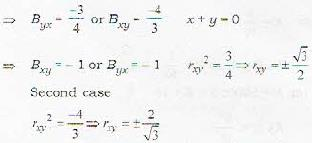Courses

# Test: Probability And Statistics - 3

## 20 Questions MCQ Test Topic-wise Tests & Solved Examples for IIT JAM Mathematics | Test: Probability And Statistics - 3

Description
This mock test of Test: Probability And Statistics - 3 for Mathematics helps you for every Mathematics entrance exam. This contains 20 Multiple Choice Questions for Mathematics Test: Probability And Statistics - 3 (mcq) to study with solutions a complete question bank. The solved questions answers in this Test: Probability And Statistics - 3 quiz give you a good mix of easy questions and tough questions. Mathematics students definitely take this Test: Probability And Statistics - 3 exercise for a better result in the exam. You can find other Test: Probability And Statistics - 3 extra questions, long questions & short questions for Mathematics on EduRev as well by searching above.
QUESTION: 1

### A speaks truth 3 times out of 4 and B speaks 7 times out of 10. They both assert that a white ball has been drawn from a bag containing 6 different colour balls. Find the probability of the truth of the assertion

Solution: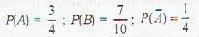If A has asserted that white ball is drawn. If white ball is drawn then he speaks truth else, he is lying.
Similar is the case with B.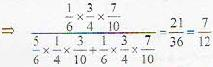i.e. (P(W, T, T) / P[W,T, T,) + P(N, F. F))

QUESTION: 2

### In four throws with a pair of dice, what is the chance of throwing doublets at least twice?

Solution:

There as 6 doublets in throw of pair of dice i.e. (1, 1), (2, 2), (3,3), (4, 4), (5, 5), (6, 6)  in 36 different types of throws.
So probability of getting a doublet = 6/36 = 1/6 and probability of not getting a doublet = 5/6. So probability of getting at least two doublets in 4 throws.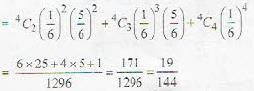QUESTION: 3

### A system composed of n separate components is said to be parallel system if it functions when at least one of the components functions. For such a system, if a component i functions with probability pi, independent of other components, i = 1, 2,....n, what is the probability that the system functions?

Solution:

Probability that the system function = probability that at least one component functions - 1- probability that none of the component functions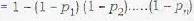QUESTION: 4

The last three digits of a telephone number have been erased and all we know is that the number was 25785???. Assuming that all possibilities are equally likely, the probability that the missing digits are all equal to each other is

Solution:

000, 111, ....999
there are 10 cases in our favour out of 10 x 10 x 10 possible case, So, probability is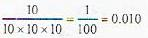QUESTION: 5

If the letters of the word REGULATION' be arranged at random, the probability that there will be exactly 4 letters between R and E is

Solution:

R E G U L A T IO N
It has 10 letters. Four letters are there between R and E, if there positions are
1, 6; 2, 7; 3, 8; 4, 9 or 5, 10 i.e. 5 ways
So, required probability is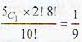QUESTION: 6

You have three coins in your pocket, two fair ones but the third biased with probability of heads p and tails 1 - p. One coin selected at random drops to the floor, landing heads up. How likely is it that it is one of the fair coins?

Solution:

One coin is selected at random and is thrown with head landing up.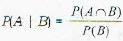By Bayes theorem for conditional probability
A : event that fair coin was selected
B : head has occurred
then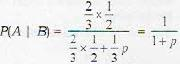QUESTION: 7

If A ∩ B = φ and B ∩ C = φ, then P(A∪B∪C) =

Solution: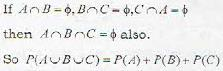QUESTION: 8

A point P is chosen at random on a line AB of length 2l. The probability P[(AP x BP) > R/2] is

Solution: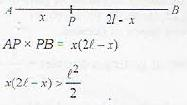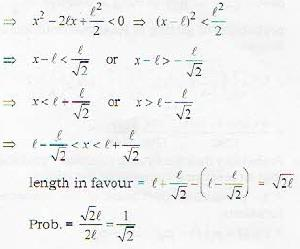QUESTION: 9

An ordinary deck of 52 playing cards is randomly divided into 4 piles of 13 cards each. The probability that each pile has exactly 1 ace is

Solution: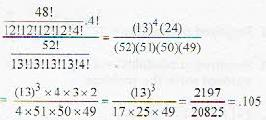QUESTION: 10

An infinite sequence of independent trials is to bo performed. Each Trial results in success with probability p and a failure with probability 1 - p. What is the probability that at least one success occurs in the first n trials?

Solution:

1 - probability of no success in n trials = 1- (1 - p)n

QUESTION: 11

Two towns A and B are 60 km apart. A school is to be built, to serve 150 students from town A and 50 students from town B. If the total distance travelled by all 200 students is to be as small as possible, then the school should be built at

Solution: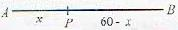If x is the distance covered by student of town A, then total distance covered by all students = 150 * x + 50 (60 - x) = 3000 + 100x
It is minimum if x = 0
So, school should be in town A.

QUESTION: 12

A random variate has the following distribution: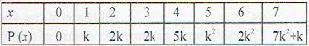The value of k is

Solution:

For random variate ∑p(x) = 1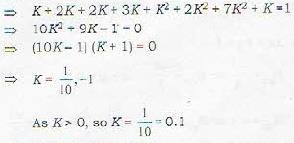QUESTION: 13

The voltage across a register is a random variable E uniform between 5V and 10 V. given the resistance of the register R = 1000 Ω, the probability density function of the power W = E2/R dissipated in R is

Solution: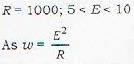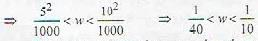For p.d.f. integration over the given interval will be 1.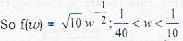QUESTION: 14

A random variate X has the following p.m.f.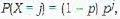j = 0, 1, 2,...
0 < p < 1
for any two positive integers m and n P(X > m + n | X > m) equals

Solution: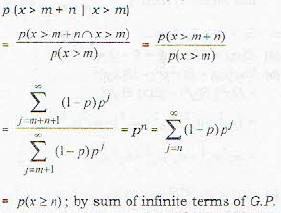QUESTION: 15

If an m / m / 1 queue, the inter-arrival time distribution is

Solution:

Arrival rate follows poisson distribution. While inter-arrival time distribution is exponential.

QUESTION: 16

Suppose that the number of typographical errors on a single page of a certain book has a Poisson distribution with parameter λ = 1/2. In 600 pages book, the average number of errors in the book is

Solution:

Mean or average of poisson distribution equals its parameter λ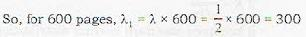QUESTION: 17

If Xand X2 are independent normal random variables with parameters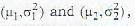respectively then X1 - X2 is normal with mean μ and variance σsuch that

Solution: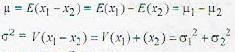QUESTION: 18

Let λ be a constant. Then var (λ X) = k var X, where k equals

Solution: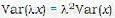⇒ K = λ2

QUESTION: 19

The weighted arithmetic mean of the first n natural numbers whose weights are equal to the corresponding numbers is given by

Solution:

Weighed Arithmetic mean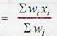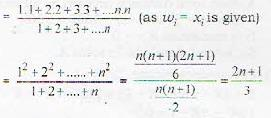QUESTION: 20

In a bivariate distribution, the lines of regression are 3x - 4y = 1 and x + y = 0, then the correlation coefficient rxy is

Solution:

3a + 4y =1